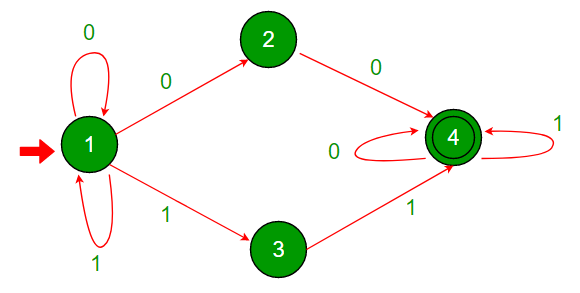C program to simulate Nondeterministic Finite Automata (NFA)

• Difficulty Level : Hard
• Last Updated : 23 Dec, 2020

Background

An NFA is typically described using a directed graph. Each edge and vertex is labeled either 0 or 1 representing possible transitions. Vertices represent the states of the NFA. Those labeled 0 are non­ accepting states, and those labeled 1 are accepting states.

• It takes an input string of finite length. Typically, the input string is a binary sequence of 0’s and 1’s.
• We begin with vertex 1 which is always the start state and follow the edges sequentially given by the bits of an input string until the input string has no further bit.
• The term ‘non ­deterministic’ means that at any state V we have more than one choice of following an edge. The NFA consumes the input string and the set of states Q represents the possible moves of NFA.
• If Q contains at least one state where the last vertex is accepting then we say that the NFA has accepted the input string otherwise the NFA has rejected the input string.
• Every NFA is not DFA, but each NFA can be translated into DFA.

Example of NFA:Starting state - vertex 1
Accepting states - Vertices with double circles(label 1) // Vertex 4
Non ­accepting states - single circles (label 0). // Vertices 1, 2 and 3.

How to check for acceptance of a string?

For Input : 1010

• In-state 1, we have two possibilities, either follow the self-loop and stay in state 1 or follow the edge labeled 1 and go to state 3.

{1} 1010 --> {1, 3} 010

• In-state 3, there is no edge labeled 0, so the computation will die out.
• In-state 1, we have two possibilities, either follow the self-loop and stay in state 1, or follow the edge labeled 0 to state 2.

{1, 3} 010 --> {1, 2} 10

• Now there is no edge labeled 1 from state 2. The computation will die out. We have two possibilities: either follow the self-loop to state 1 or follow the edge labeled 1 to state 3.

{1, 2} 10 --> {1, 3} 0

• In-state 3, there is no edge labeled 0. So the computation will die out. In-state 1, we have two possibilities: either follow the self-loop to state 1 or the edge labeled 0 to state 2.

{1, 3} 0 --> {1, 2}

• Now the NFA has consumed the input. It can be either be in states 1 or 2, both of which are non ­accepting. So the NFA has rejected the input 1010.

For Input: 1100

{1} 1100 --> {1, 3} 100 {1, 3} 100 --> {1, 3, 4} 00 {1, 3, 4}
00--> {1, 2, 4} 0 {1, 2, 4} 0--> {1, 2, 4}

• Now the NFA has consumed the input. It can either be in states 1, 2, or 4. State 4 is an accepting state. So, the NFA accepts the string 1100.
• We can easily verify that the given NFA accepts all binary strings with “00” and/or “11” as a substring.

C Program to simulate Nondeterministic Finite Automata (NFA)

Input Format:  The adjacency list representation of the NFA is in the following format.
The given example will be represented as
Total Number of Edges: 4
Edge Connectivity:
1 0 4 0 1 0 2 1 1 1 3
2 0 1 0 4
3 0 1 1 4
4 1 2 0 4 1 4

Output Format:  The first 10 binary strings which are accepted by the NFA  in lexicographical order (e denotes the empty string): {e, 0, 1, 00, 01, 10, 11, 000, …}

The sample output for the given test case is as follows:
00
11
000
001
011
100
110
111

C

 #include #include #include #include #include  int row = 0; // A structure to represent an adjacency list nodestruct node{    int data;    struct node* next;    char edgetype; }typedef node; // Adds an edge to an adjacency listnode* push(node* first , char edgetype , int data){    node* new_node = (node*)malloc(sizeof(node));    new_node->edgetype = edgetype;    new_node->data = data;    new_node->next = NULL;    if (first==NULL)    {        first = new_node;        return new_node;    }    first->next = push(first->next,edgetype,data);    return first;} //Recursive function to check acceptance of inputint nfa(node** graph, int current, char* input,        int* accept, int start){    if (start==(int)strlen(input))        return accept[current];     node* temp = graph[current];    while (temp != NULL)    {      if (input[start]==temp->edgetype)        if (nfa(graph,temp->data,input,accept,start+1==1))           return 1;      temp=temp->next;    }    return 0;} //Function to generate binary strings of size nvoid generate(char** arr, int size, char *a){    if (size==0)    {        strcpy(arr[row], a);        row++;        return;    }    char b0 = {'\0'};    char b1 = {'\0'};    b0 = '0';    b1 = '1';     //Recursively generate the binary string    generate((char**)arr, size-1, strcat(b0,a)); //Add 0 in front    generate((char**)arr, size-1, strcat(b1,a)); //Add 1 in front    return; } // Driver program to test above functionsint main(){    int n;    int i, j;    scanf("%d", &n); //Number of nodes    node* graph[n+1]; //Create a graph     for (i=0;i

Input:

4
1 0 4 0 1 0 2 1 1 1 3
2 0 1 0 4
3 0 1 1 4
4 1 2 0 4 1 4

Output:

00
11
000
001
011
100
110
111
0000
0001